# Calculus 1 Part 1 - Limits and Derivatives

Teacher: Cheryl
Customers Who Have Viewed This Course: 8451
\$200.00

#### 0 Introduction 03:05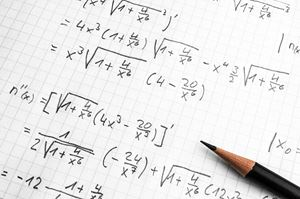This video will tell you about Calculus 1 Part 1, explaining the topics covered (Limits and Differentiation), what comes with each lesson (a 10-30 minute video lecture, a short self-grading test, a practice worksheet, a video going over problems from the practice worksheet, and a solution sheet in the resources section), what you should be familiar with before starting (Algebra and a bit of Trig), and welcoming you to the class!

#### 1 Finding Limits Graphically 18:34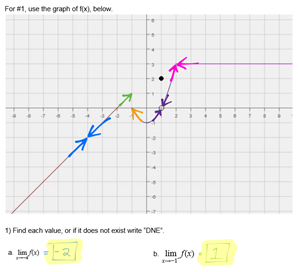This lesson introduces the concept of limits, then demonstrates how to find limits from graphs in several examples.  We go over limits at continuous points, left- and right-limits, and limits involving asymptotes, both vertical and horizontal.  This is very much a visual lesson, with little actual math needed, but it is important to understand the graphical interpretation of limits to thrive in Calculus!

The lesson includes a video lecture, a quick test of your understanding, a worksheet, and a solution sheet in the resources section.  It is important to do the worksheet, and not just take the self-grading test!  After completing the worksheet, check your answers against the solution sheet in the resource folder.

This worksheet is multiple pages long, but don't worry, that's just because of the large graphs!  Most of the worksheets in this class will be a single page.  There is also only one worksheet for this lesson, despite what the end of the video says!

#### 2 Worksheet Review: Finding Limits Graphically 10:24

This video will go over the practice worksheet, demonstrating step-by-step solutions to problems from the worksheet.

#### 3 Finding Limits Algebraically 13:49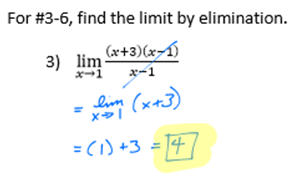This lesson will demonstrate the two most common methods for finding limits algebraically: plugging in, and reducing.

To fully complete this lesson, you will need to know how to factor quadratics.  Several of the homework problems and lecture examples require factoring.

This lesson includes a video lecture, a self-grading test, a practice worksheet, and a solution sheet in the resources section.

#### 4 Worksheet Review: Finding Limits Algebraically 20:05

This video will go over the practice worksheet, demonstrating step-by-step solutions to problems from the worksheet.

#### 5 Finding Limits Approaching Asymptotes Algebraically 20:33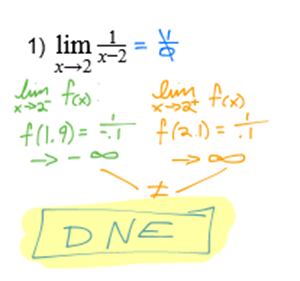This lesson covers how to find the limit of a function as x approaches a vertical asymptote, without graphing.

The lesson includes a video lecture, a short self-grading test, a practice worksheet, and a solution sheet in the resources section.  It is important to do the practice worksheet, as the self-grading test will not thoroughly check your mastery of this lesson.

In the video, the first example is done first with a graph, and then again without a graph, using test points.  The graph is used to aid comprehension of the concept only; you should not use graphs in your homework!  The second example uses tables, which is a method covered in many classes, though it can be significantly more time consuming than taking single test points.

I recommend having a calculator on hand when you do the worksheet with this lesson.  Do not use it to graph the function!  There are a lot of decimals involved with these problems, so a calculator will save you a lot of time and effort.

#### 6 Worksheet Review: Finding Limits Approaching Asymptotes Algebraically 29:11

This video will go over the practice worksheet, demonstrating step-by-step solutions to problems from the worksheet.

#### 7 Finding Limits Approaching Infinity Algebraically 19:03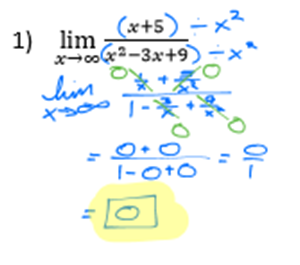This lesson covers how to find limits approaching positive or negative infinity, using non-graphical, algebraic methods.

The lesson includes a video lecture, a short self-grading test, a practice worksheet, and a solution sheet in the resources section.  It is important to do the practice worksheet, as the self-grading test will not thoroughly check your mastery of this lesson.

If you remember learning to find horizontal asymptotes of rational functions, this may be an easy lesson for you, as the concepts are very closely related!

In this video I covered a very formal method, but there are several approaches for finding horizontal asymptotes.  There is a short-cut mnemonic device, "BOBO BOTN BETC", that works well for some students, which I encourage you to look up independently if the methods demonstrated in the video do not seem completely clear to you.

#### 8 Worksheet Review: Finding Limits Approaching Infinity Algebraically 21:04

This video will go over the practice worksheet, demonstrating step-by-step solutions to problems from the worksheet.

I say in the beginning of this video that this is for limits approaching asymptotes, but this is the correct video, for limits approaching infinity!

#### 9 Some Common Limits 16:44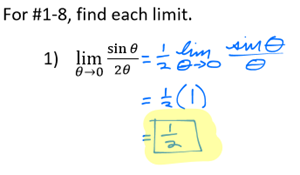This lesson will cover some common limits and limit types that you're likely to run into, and demonstrates how to solve them.

This lesson includes a video lecture, a short self-grading test, a practice worksheet, and a solution sheet in the resources section.  It is important to do the practice worksheet, as the self-grading test will not thoroughly check your mastery of the lesson.

These limits are common enough to be worth memorizing, especially if you are preparing for an AP test.  Whatever level of Calculus you are taking, I strongly recommend putting together a "cheat sheet" of rules and formulas you will need throughout the class, so you can quickly refer back to these.  Many of your practice worksheets throughout this class will have a Resources box, like this practice worksheet does, with handy rules or formulas.  You should consider putting anything in these boxes in your "cheat sheet", and/or memorizing them.

#### 10 Worksheet Review: Some Common Limits 13:51

This video will go over the practice worksheet, demonstrating step-by-step solutions to problems from the worksheet.

#### 11 Squeeze Theorem 16:43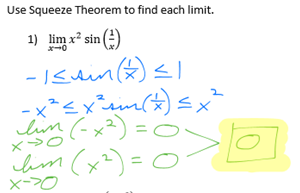This lesson will explain the concept behind Squeeze Theorem, and demonstrate several problems using it.

This lesson includes a video lecture, a short self-grading test, a practice worksheet, and a solution sheet in the resources section.  It is important to do the practice worksheet, as the self-grading test will not thoroughly check your mastery of the lesson.

Squeeze Theorem is one of the more advanced concepts related to limits, and some non-AP class map skip over it entirely.  However, it is a useful concept to be familiar with, and an interesting application of limits!

#### 12 Worksheet Review: Squeeze Theorem 13:51

This video will go over the practice worksheet, demonstrating step-by-step solutions to problems from the worksheet.

#### 13 Continuity 20:39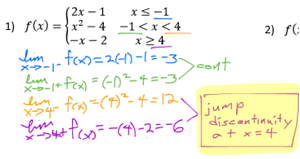This lesson will explain the concept of continuity and the different types of discontinuity.  You will learn to find discontinuities and identify their type.  Both graphical and algebraic explanations will be used in the lecture.

This lesson includes a video lecture, a short self-grading test, a practice worksheet, and a solution sheet in the resources section.  It is important to do the practice worksheet, as the self-grading test will not thoroughly check your mastery of the lesson.

This lesson will include a few piecewise functions, as well as vertical asymptotes of rational functions, so you may want to review either or both of those topics if it has been a while.

#### 14 Worksheet Review: Continuity 17:50

This video will go over the practice worksheet, demonstrating step-by-step solutions to problems from the worksheet.

#### 15 Limits Test 01:04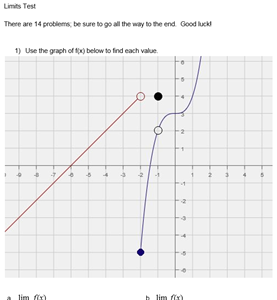This test will check your understanding of everything we've covered in the Limits section.

The solution sheet is in the Resources section.

If you are preparing for an AP exam or similar test, you should consider this test close-book, closed-notes.

Otherwise, you can use a single "cheat sheet", with rules and formulas you think you might need.  I'd recommend that anything in a Reference box at the top of a practice worksheet be either memorized or on your "cheat sheet".

You may use a graphing calculator on this test.

#### 16 The Difference Quotient 29:52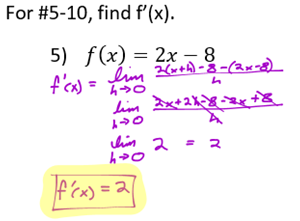This lesson will cover the difference quotient, and how to use it to find derivatives.

This lesson includes a video lecture, a short self-grading test, a practice worksheet, and a solution sheet in the resources section.  It is important to do the practice worksheet, as the self-grading test will not thoroughly check your mastery of the lesson.

The difference quotient is the basis for finding all derivatives, though most of this section of the class will be devoted to learning short cuts for different types of functions.  In this lecture we discuss what a derivative is, and why the difference quotient works.  However, I would not worry too much about this for now!  You will not really need to understand what a derivative is, or what it means, or why it matters, until you get to Applications of Derivatives.  For now, focus on mastering finding derivatives.  This video will include several examples of using the difference quotient to find derivatives of given functions.

#### 17 Worksheet Review: The Difference Quotient 26:26

This video will go over the practice worksheet, demonstrating step-by-step solutions to problems from the worksheet.

#### 18 Basic Derivatives 20:48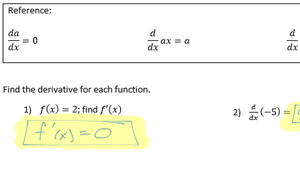This lesson introduces the short cut for finding the derivatives of basic polynomials, laying the ground work for many of the methods we will be covering in this course.

This lesson includes a video lecture, a short self-grading test, a practice worksheet, and a solution sheet in the resources section.  It is important to do the practice worksheet, as the self-grading test will not thoroughly check your mastery of the lesson.

As discused in the last lesson, most of this class will actually be learning short-cut methods to find derivatives.  This method works for polynomial functions, and is very quick and easy!  Make sure you're comfortable with it before moving forward, as you will be using it a lot througout Calculus!

#### 19 Worksheet Review: Basic Derivatives 08:36

This video will go over the practice worksheet, demonstrating step-by-step solutions to problems from the worksheet.

#### 20 Basic Derivatives Part 2 19:20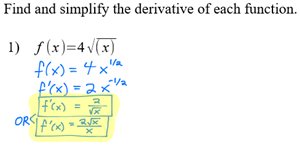This cover some less obvious applications of the methods learned in "Basic Derivatives".

This lesson includes a video lecture, a short self-grading test, a practice worksheet, and a solution sheet in the resources section.  It is important to do the practice worksheet, as the self-grading test will not thoroughly check your mastery of the lesson.

This is essentially the same method as the last lesson, but includes negative and fraction exponents.

#### 21 Worksheet Review: Basic Derivatives Part 2 18:01

This video will go over the practice worksheet, demonstrating step-by-step solutions to problems from the worksheet.

#### 22 Chain Rule 26:20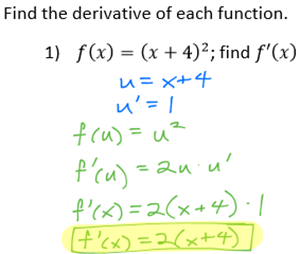This lesson covers Chain Rule, a very important method to master for taking derivatives.

This lesson includes a video lecture, a short self-grading test, a practice worksheet, and a solution sheet in the resources section.  It is important to do the practice worksheet, as the self-grading test will not thoroughly check your mastery of the lesson.

The Chain Rule will be used constantly throughout Calculus, and is where many students begin to struggle.  If you are prone to writing your work in an all-over-the-page style, now is a good time to cultivate a neater, more formal system.  Clearly write each step.  You may be able to skip some steps, and that's fine, but everything you write should be clearly labeled, with an equal sign, not just floating there.  Indicate what a string of numbers and variables equals, rather than just leaving it there - is it u, u', f(x), f(u), f'(x), f'(u), what?  It's important to keep track.  I cannot stress this enough.  Many very bright students are able to get this far in math without developing a neat style for keeping track of their work, because they're able to track it all in their head, and this is where it starts to catch up with them!  From here on, Calculus problems can get very messy, with many parts.  It is very important to keep track of which parts are what, so you don't make small mistakes and ruin an entire problem.  The problems in this lesson are relatively simple, compared to some we'll be getting to, so use this lesson to get into the habit of writing work neatly.

#### 23 Worksheet Review: Chain Rule 25:54

This video will go over the practice worksheet, demonstrating step-by-step solutions to problems from the worksheet.

#### 24 More Derivative Rules 20:00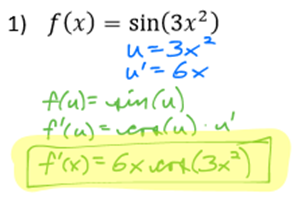This lesson covers many derivative rules that you will need to know as you continue through Calculus.

You will need to use Chain Rule to solve these problems.  Assume you will need Chain Rule for the rest of Calculus!

This lesson includes a video lecture, a short self-grading test, a practice worksheet, and a solution sheet in the resources section.  It is important to do the practice worksheet, as the self-grading test will not thoroughly check your mastery of the lesson.

These are some common function types you will need to be able to take derivatives of throughout Calculus.  The worksheet includes a reference box, and you should add these rules to your "cheat sheet", or memorize them, as you will need them throughout Calculus.

#### 25 Worksheet Review: More Derivative Rules 24:37

This video will go over the practice worksheet, demonstrating step-by-step solutions to problems from the worksheet.

#### 26 Product and Quotient Rules 27:23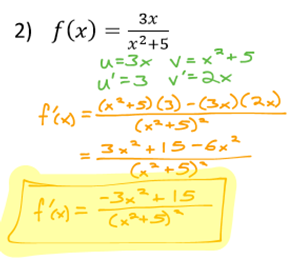This lesson introduces the Product Rule and Quotient Rule, which are both very commonly used in Calculus.

You will need Chain Rule and the rules from "More Derivative Rules" for this lesson.  Again, assume you will need these for the rest of Calculus!

This lesson includes a video lecture, a short self-grading test, a practice worksheet, and a solution sheet in the resources section.  It is important to do the practice worksheet, as the self-grading test will not thoroughly check your mastery of the lesson.

The Product Rule and Quotient Rule are very common techniques for taking derivatives of functions that would otherwise be very difficult to work with.  Note that as we continue through this class, each new lesson will require what you learned in the last one.

The lessons covered so far, from Basic Derivatives to this one, are what I would consider the basics of differentiation.  Make sure you have mastered these skills!

#### 27 Worksheet Review: Product and Quotient Rules 29:38

This video will go over the practice worksheet, demonstrating step-by-step solutions to problems from the worksheet.

#### 28 Derivatives Test 1 01:02

This test will check your understanding of everything in this section so far, lessons "The Difference Quotient" to "Product and Quotient Rules"

The solution sheet is in the Resources section.

If you are preparing for an AP exam or similar, your should take this test closed-book, closed-notes.

Otherwise, you should have a single "cheat sheet" with rules or formulas you might need.  I recommend anything that's been in a Reference box as the top of a practice worksheet be either memorized or included on your "cheat sheet".

You may use a graphing calculator or regular calculator on this test.

#### 29 Second and Third Derivatives 29:17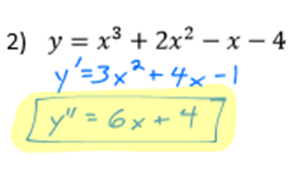This introduces the concept of higher order derivatives.

This lesson includes a video lecture, a short self-grading test, a practice worksheet, and a solution sheet in the resources section.  It is important to do the practice worksheet, as the self-grading test will not thoroughly check your mastery of the lesson.

Second and third derivatives are pretty much exactly what they sound like, there are really no new skills in this lesson!  Consider this a good opportunity to practice the skills you've already learned, while mastering a new concept.

#### 30 Worksheet Review: Second and Third Derivatives 29:50

This video will go over the practice worksheet, demonstrating step-by-step solutions to problems from the worksheet.

#### 31 Derivatives at Exact Values 24:46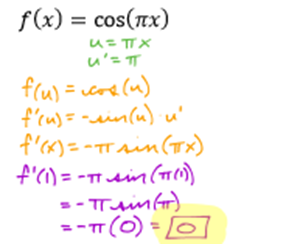This covers how to find derivatives and second derivatives at exact x-values.

This lesson includes a video lecture, a short self-grading test, a practice worksheet, and a solution sheet in the resources section.  It is important to do the practice worksheet, as the self-grading test will not thoroughly check your mastery of the lesson.

This is another pretty easy lesson, and more good practice taking derivatives!

#### 32 Worksheet Review: Derivatives at Exact Values 25:07

This video will go over the practice worksheet, demonstrating step-by-step solutions to problems from the worksheet.

#### 33 Inverse Trig Derivatives 19:38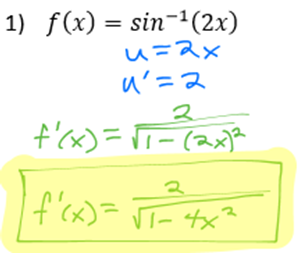This introduces the rules for taking the derivatives of inverse trig functions.

This lesson includes a video lecture, a short self-grading test, a practice worksheet, and a solution sheet in the resources section.  It is important to do the practice worksheet, as the self-grading test will not thoroughly check your mastery of the lesson.

The derivatives rules for inverse trig functions can be intimidating for some students, as they have some fairly large, messy fractions and radicals, but don't let that overwhelm you!  These rules are large, but not really any more difficult than any of the rules you learned in "More Derivative Rules".  As additional good news, you don't really use them much!  I would include these rules on your "cheat sheet", but if you are not preparing for an AP or similar exam, I wouldn't bother memorizing them, and some classes may even skip them entirely.  Nevertheless, they are good to be familar with.

#### 34 Worksheet Review: Inverse Trig Derivatives 29:30

This video will go over the practice worksheet, demonstrating step-by-step solutions to problems from the worksheet.

#### 35 Implicit Differentiation 19:04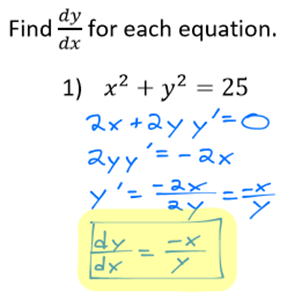This introduces the implicit differentiation method.

This lesson includes a video lecture, a short self-grading test, a practice worksheet, and a solution sheet in the resources section.  It is important to do the practice worksheet, as the self-grading test will not thoroughly check your mastery of the lesson.

Implicit Differentiation is less commonly used than most of the methods we've covered in this class, but important to master for later Calculus.  You will definitely be using it in Applications of Derivatives, especially word problems involving optimization.  If you are taking any kind of Physics or Engineering classes, or plan to be in the future, this is a technique that will come in handy there as well.

This can be one of the more difficult concepts for some students, and is pretty Algebra-heavy.  Take your time and go through the lesson carefully.

#### 36 Worksheet Review: Implicit Differentiation 28:28

This video will go over the practice worksheet, demonstrating step-by-step solutions to problems from the worksheet.

#### 37 Derivatives Test 2 00:52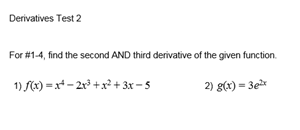This will test your understanding of the lessons from "Second and Third Derivatives" to "Implicit Differentiation".

The solution sheet is in the Resources section.

If you are preparing for an AP exam or similar, you should take this test closed-book, closed-notes.

Otherwise, you should have a single "cheat sheet" with any rules or formulas you might need.  I recommend including anything from a Reference box on the top of a practice worksheet.

You may use a graphing calculator on this test.

#### 38 Final Exam 00:53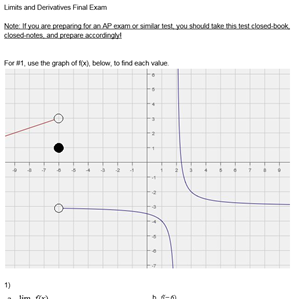This is the final exam for the entire class, Limits and Differentiation.

As usual, the solution sheet is in the resources section.

You may use a graphing calculator on this test.

If you are preparing for an AP exam, or similar, you should consider this a closed-book, closed-notes test!

Otherwise, you can have a single "cheat sheet" with useful rules and formulas.

I recommend that everything that has been is a Feference box at the top of a practice worksheet be either memorized or on your cheat sheet.  You will need to know those rules.

Calculus 1 Part 1 – Limits and Differentiation

This is not a full Calculus I class! This course covers finding limits and taking derivatives, the first things covered in most Calculus classes.  I will be putting out a full Calculus series over the next few months, which will cover everything a typical highschool AP Calculus AB class would cover.  It's sold in sections for the convenience of students who only need help with part of Calculus.

This course can be used as a stand alone class, to prepare for an AP Calculus exam or other Calculus test, or to supplement most Calculus I classes.

Course Objective

Develop and master the ability to find limits and derivatives.

Course Description

Calculus 1 Part 1 covers limits and differentiation. The concept of limits will be explored both algebraically and graphically. Multiple methods for finding limits will be explored and demonstrated, with many example problems.

Next, the course covers differentiation, or finding derivatives. We'll briefly cover what a derivative is; what it means both algebraically and graphically. Most of the Differentiation section, however, is focused on the mathematical process of finding derivatives, covering many different techniques and rules you will need to become proficient at differentiation. A more thorough understanding of the concept and applications of derivatives will be covered in the next course, Applicationations of Differentiation.

Course content

• 17 lessons
• over 12 hours of video
• a video lecture with each lesson
• short self-grading test for each lesson
• practice worksheets for each lesson
• a review video for each practice worksheet
• full solution sheet for each practice worksheet
• three progress tests, with solution sheets
• a final exam, with a solution sheet

Suggested Audience

This course is based on AP Calculus AB material, usually taken in the 12th grade, and comparable to a college Calculus 1 class. If you are preparing for an AP exam, preparing for college Calculus, or supplementing any high school Calculus class, this is the right course series for you!

If you are preparing for an AP Calculus BC exam, or preparing for Engineering Calculus in college, this course series will cover most but not all of the topics you will need to master.

Requirements

Algebra I and II, Geometry, Precalculus

A strong Algebra fluency is most important. You should also have a basic understanding of Trigonometry.

I also recommend a good graphing calculator!  A TI-83 or TI-84 is best to be familar with if you're preparing for any sort of standardized test, as that's what you'll be allowed to use during the test (Typically.  Check what your exact test allows!).  If you're not preparing for a standardized test, there are a lot of good free graphing calculator apps out there.

• Teacher: Cheryl
• Areas of expertise: I have experience teaching and tutoring all high school math, but especially enjoy Calculus and Precal!
• Education: I have a BS in Geoscience from UT Dallas, and am an experienced Texas certified teacher.
• Interests: I enjoy math, running, and am attempting to learn Danish!
• Skills: I can solve any size rubiks cube. I'm hip like that.
• Associations: Mensa member
• Issues I care about: Pi vs Tau. Tau makes so much more sense than Pi!

I've been teaching and tutoring since I graduated from college in 2008, and teaching math specifically since 2011. I have taught ESL in South Korea, taught at a Texas public school, tutored privately for high school and college math, taught high school math in a private school for learning different students, and am now very excited to be teaching through Lernsys!

#### Finding Limits Graphically solution sheet

This is the solution sheet for the "Finding Limits Graphically" practice worksheet.

#### Finding Limits Algebraically solution sheet

This is the solution sheet for the "Finding Limits Algebraically" practice worksheet.

#### Finding Limits Approaching Asymptotes Algebraically solution sheet

This is the solution sheet for the "Finding Limits Approaching Asymptotes Algebraically" practice worksheet.

#### Finding Limits Approaching Infinity Algebraically solution sheet

This is the solution sheet for the "Finding Limits Approaching Infinity Algebraically" practice worksheet.

#### Some Common Limits solution sheet

This is the solution sheet for the "Some Common Limits" practice worksheet.

#### Squeeze Theorem solution sheet

This is the solution sheet for the "Squeeze Theorem" practice worksheet.

#### Continuity solution sheet

This is the solution sheet for the "Continuity" practice worksheet.

#### Limits Test solution sheet

This is the solution sheet for the Limits Test.

#### The Difference Quotient solution sheet

This is the solution sheet for the "The Difference Quotient" practice worksheet.

#### Basic Derivatives solution sheet

This is the solution sheet for the "Basic Derivatives" practice worksheet.

#### Basic Derivatives Part 2 solution sheet

This is the solution sheet for the "Basic Derivatives Part 2" practice worksheet.

#### Chain Rule solution sheet

This is the solution sheet for the "Chain Rule" practice worksheet.

#### More Derivative Rules solution sheet

This is the solution sheet for the "More Derivative Rules" practice worksheet.

#### Product and Quotient Rules solution sheet

This is the solution sheet for the "Product and Quotient Rules" practice worksheet.

#### Derivative Test 1 solution sheet

This is the solution sheet for Derivative Test 1.

#### Second and Third Derivatives solution sheet

This is the solution sheet for the "Second and Third Derivatives" practice worksheet.

#### Derivatives at Exact Values solution sheet

This is the solution sheet for the "Derivatives at Exact Values" practice worksheet.

#### Inverse Trig Derivatives solution sheet

This is the solution sheet for the "Inverse Trig Derivatives" practice worksheet.

#### Implicit Differentiation solution sheet

This is the solution sheet for the "Implicit Differentiation" practice worksheet.

#### Derivatives Test 2 solution sheet

This is the solution sheet for Derivatives Test 2.

#### Limits and Derivatives Final Exam solution sheet

This is the solution sheet for the Limits and Derivatives Final Exam.